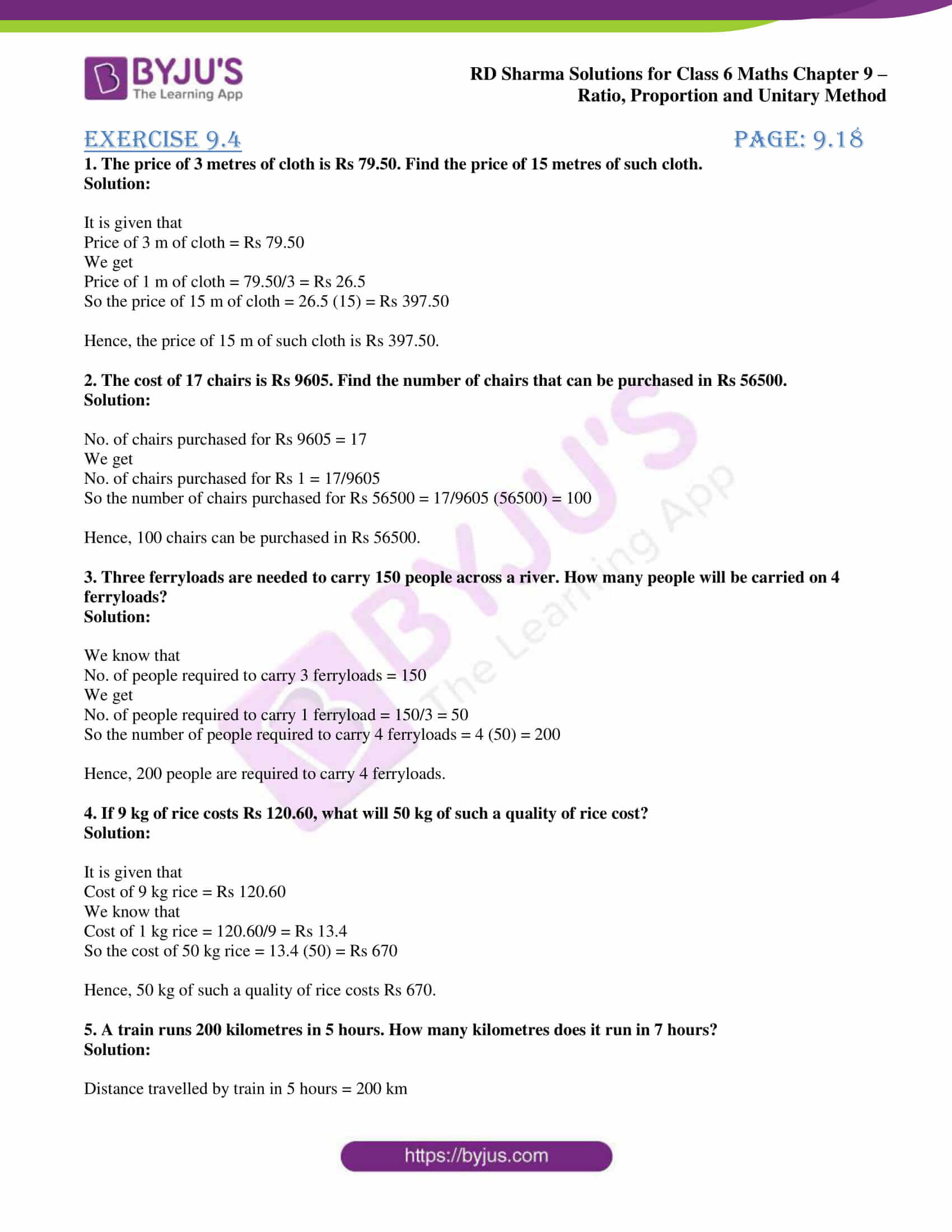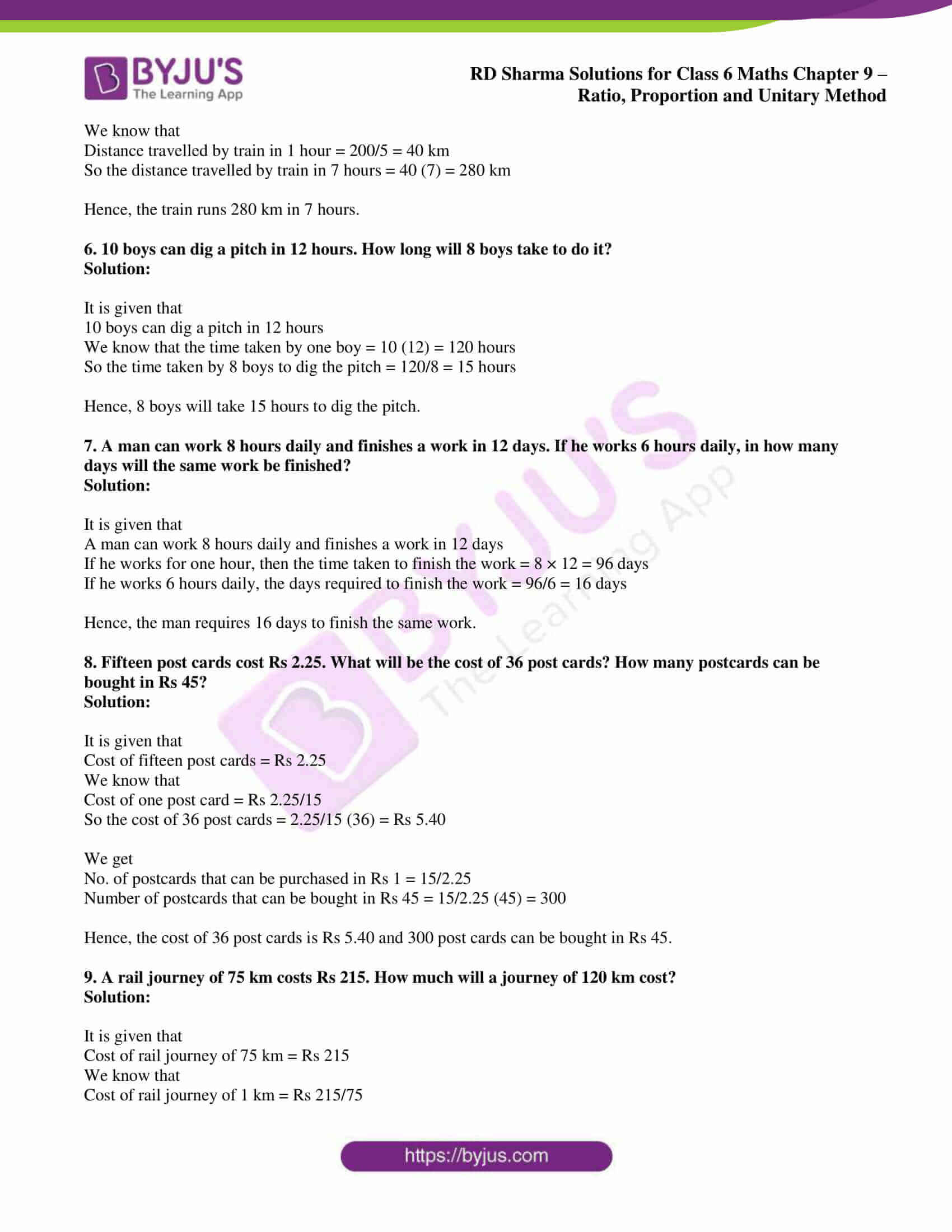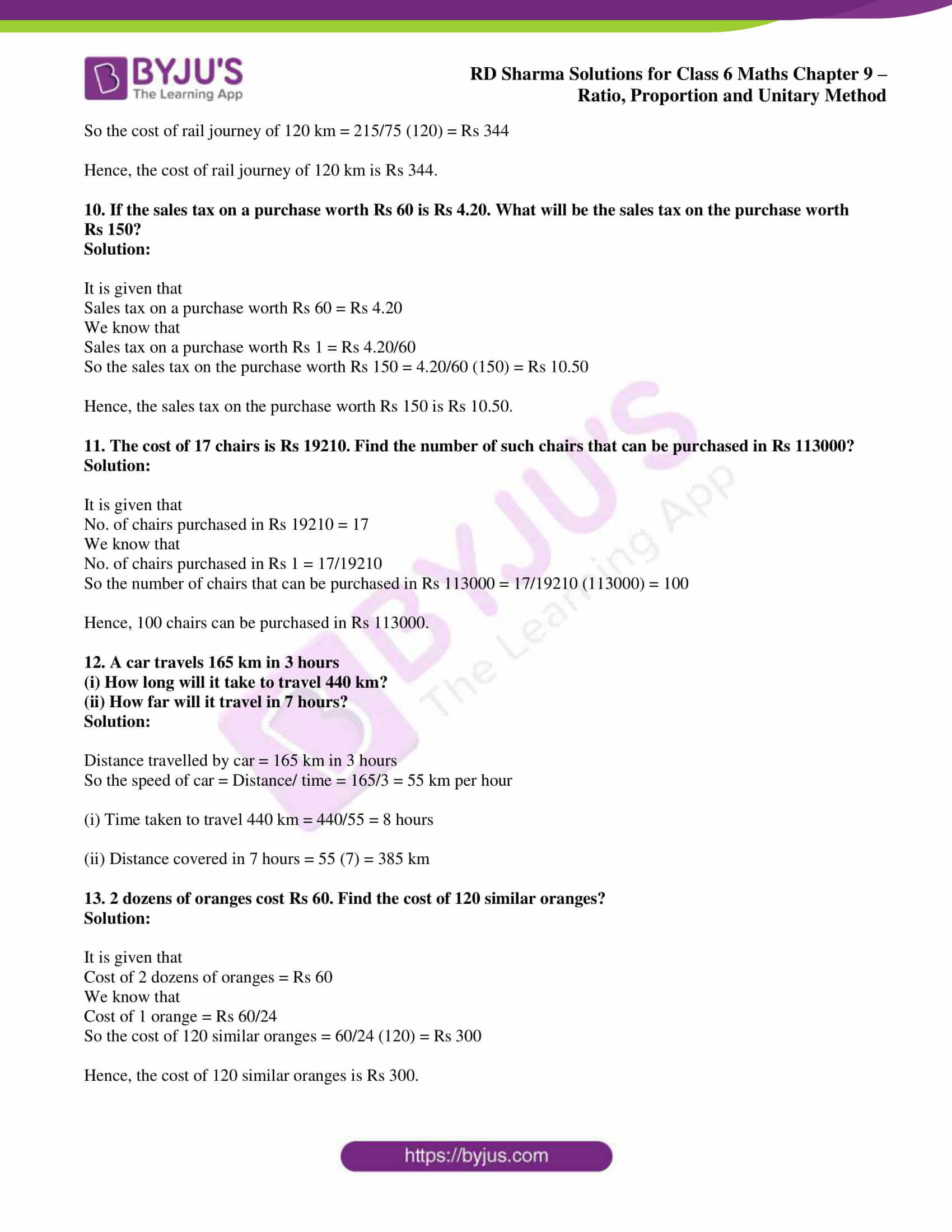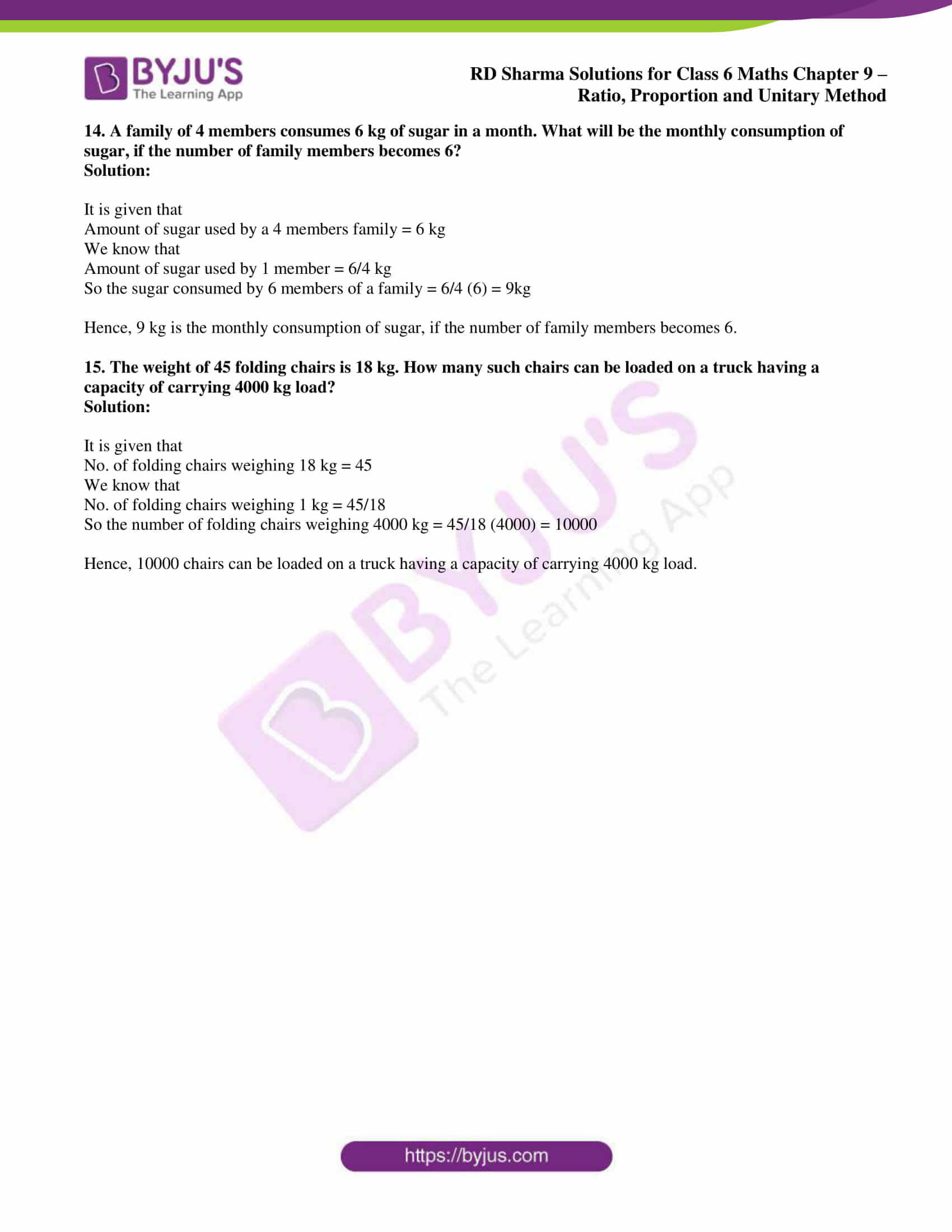# RD Sharma Solutions for Class 6 Maths Chapter 9: Ratio, Proportion and Unitary Method Exercise 9.4

Students who don’t get their doubts cleared during class hours can make learning more joyful using RD Sharma Solutions Class 6. This exercise has questions based on the unitary method. A unitary method is a method of determining the value of one unit and then of the number of units. The solutions are prepared based on the understanding capacity of students. RD Sharma Solutions for Class 6 Maths Chapter 9 Ratio, Proportion and Unitary Method Exercise 9.4 PDF are provided here.

## RD Sharma Solutions for Class 6 Maths Chapter 9: Ratio, Proportion and Unitary Method Exercise 9.4 Download PDF### Access answers to Maths RD Sharma Solutions for Class 6 Chapter 9: Ratio, Proportion and Unitary Method Exercise 9.4

1. The price of 3 metres of cloth is Rs 79.50. Find the price of 15 metres of such cloth.

Solution:

It is given that

Price of 3 m of cloth = Rs 79.50

We get

Price of 1 m of cloth = 79.50/3 = Rs 26.5

So the price of 15 m of cloth = 26.5 (15) = Rs 397.50

Hence, the price of 15 m of such cloth is Rs 397.50.

2. The cost of 17 chairs is Rs 9605. Find the number of chairs that can be purchased in Rs 56500.

Solution:

No. of chairs purchased for Rs 9605 = 17

We get

No. of chairs purchased for Rs 1 = 17/9605

So the number of chairs purchased for Rs 56500 = 17/9605 (56500) = 100

Hence, 100 chairs can be purchased in Rs 56500.

3. Three ferryloads are needed to carry 150 people across a river. How many people will be carried on 4 ferryloads?

Solution:

We know that

No. of people required to carry 3 ferryloads = 150

We get

No. of people required to carry 1 ferryload = 150/3 = 50

So the number of people required to carry 4 ferryloads = 4 (50) = 200

Hence, 200 people are required to carry 4 ferryloads.

4. If 9 kg of rice costs Rs 120.60, what will 50 kg of such a quality of rice cost?

Solution:

It is given that

Cost of 9 kg rice = Rs 120.60

We know that

Cost of 1 kg rice = 120.60/9 = Rs 13.4

So the cost of 50 kg rice = 13.4 (50) = Rs 670

Hence, 50 kg of such a quality of rice costs Rs 670.

5. A train runs 200 kilometres in 5 hours. How many kilometres does it run in 7 hours?

Solution:

Distance travelled by train in 5 hours = 200 km

We know that

Distance travelled by train in 1 hour = 200/5 = 40 km

So the distance travelled by train in 7 hours = 40 (7) = 280 km

Hence, the train runs 280 km in 7 hours.

6. 10 boys can dig a pitch in 12 hours. How long will 8 boys take to do it?

Solution:

It is given that

10 boys can dig a pitch in 12 hours

We know that the time taken by one boy = 10 (12) = 120 hours

So the time taken by 8 boys to dig the pitch = 120/8 = 15 hours

Hence, 8 boys will take 15 hours to dig the pitch.

7. A man can work 8 hours daily and finishes a work in 12 days. If he works 6 hours daily, in how many days will the same work be finished?

Solution:

It is given that

A man can work 8 hours daily and finishes a work in 12 days

If he works for one hour, then the time taken to finish the work = 8 × 12 = 96 days

If he works 6 hours daily, the days required to finish the work = 96/6 = 16 days

Hence, the man requires 16 days to finish the same work.

8. Fifteen post cards cost Rs 2.25. What will be the cost of 36 post cards? How many postcards can be bought in Rs 45?

Solution:

It is given that

Cost of fifteen post cards = Rs 2.25

We know that

Cost of one post card = Rs 2.25/15

So the cost of 36 post cards = 2.25/15 (36) = Rs 5.40

We get

No. of postcards that can be purchased in Rs 1 = 15/2.25

Number of postcards that can be bought in Rs 45 = 15/2.25 (45) = 300

Hence, the cost of 36 post cards is Rs 5.40 and 300 post cards can be bought in Rs 45.

9. A rail journey of 75 km costs Rs 215. How much will a journey of 120 km cost?

Solution:

It is given that

Cost of rail journey of 75 km = Rs 215

We know that

Cost of rail journey of 1 km = Rs 215/75

So the cost of rail journey of 120 km = 215/75 (120) = Rs 344

Hence, the cost of rail journey of 120 km is Rs 344.

10. If the sales tax on a purchase worth Rs 60 is Rs 4.20. What will be the sales tax on the purchase worth Rs 150?

Solution:

It is given that

Sales tax on a purchase worth Rs 60 = Rs 4.20

We know that

Sales tax on a purchase worth Rs 1 = Rs 4.20/60

So the sales tax on the purchase worth Rs 150 = 4.20/60 (150) = Rs 10.50

Hence, the sales tax on the purchase worth Rs 150 is Rs 10.50.

11. The cost of 17 chairs is Rs 19210. Find the number of such chairs that can be purchased in Rs 113000?

Solution:

It is given that

No. of chairs purchased in Rs 19210 = 17

We know that

No. of chairs purchased in Rs 1 = 17/19210

So the number of chairs that can be purchased in Rs 113000 = 17/19210 (113000) = 100

Hence, 100 chairs can be purchased in Rs 113000.

12. A car travels 165 km in 3 hours

(i) How long will it take to travel 440 km?

(ii) How far will it travel in 7 hours?

Solution:

Distance travelled by car = 165 km in 3 hours

So the speed of car = Distance/ time = 165/3 = 55 km per hour

(i) Time taken to travel 440 km = 440/55 = 8 hours

(ii) Distance covered in 7 hours = 55 (7) = 385 km

13. 2 dozens of oranges cost Rs 60. Find the cost of 120 similar oranges?

Solution:

It is given that

Cost of 2 dozens of oranges = Rs 60

We know that

Cost of 1 orange = Rs 60/24

So the cost of 120 similar oranges = 60/24 (120) = Rs 300

Hence, the cost of 120 similar oranges is Rs 300.

14. A family of 4 members consumes 6 kg of sugar in a month. What will be the monthly consumption of sugar, if the number of family members becomes 6?

Solution:

It is given that

Amount of sugar used by a 4 members family = 6 kg

We know that

Amount of sugar used by 1 member = 6/4 kg

So the sugar consumed by 6 members of a family = 6/4 (6) = 9kg

Hence, 9 kg is the monthly consumption of sugar, if the number of family members becomes 6.

15. The weight of 45 folding chairs is 18 kg. How many such chairs can be loaded on a truck having a capacity of carrying 4000 kg load?

Solution:

It is given that

No. of folding chairs weighing 18 kg = 45

We know that

No. of folding chairs weighing 1 kg = 45/18

So the number of folding chairs weighing 4000 kg = 45/18 (4000) = 10000

Hence, 10000 chairs can be loaded on a truck having a capacity of carrying 4000 kg load.